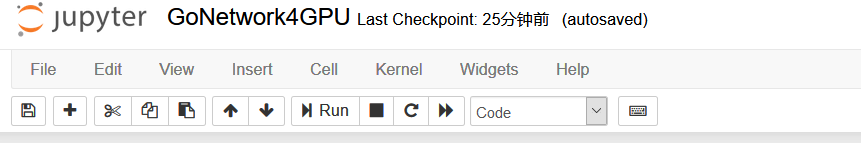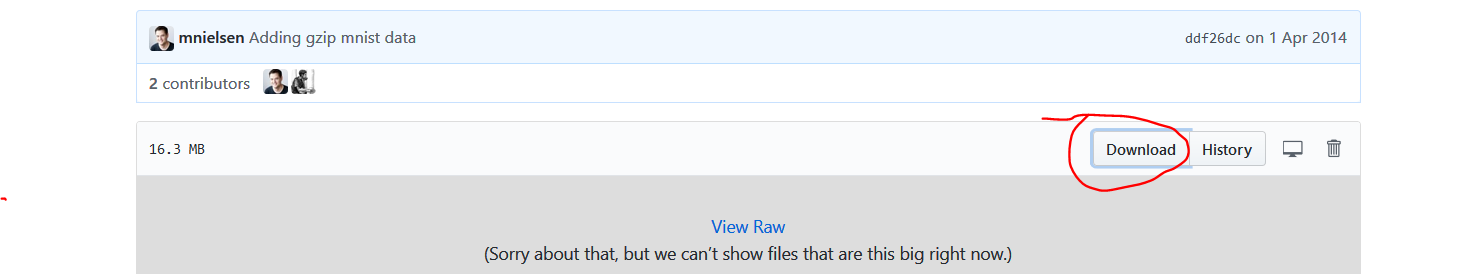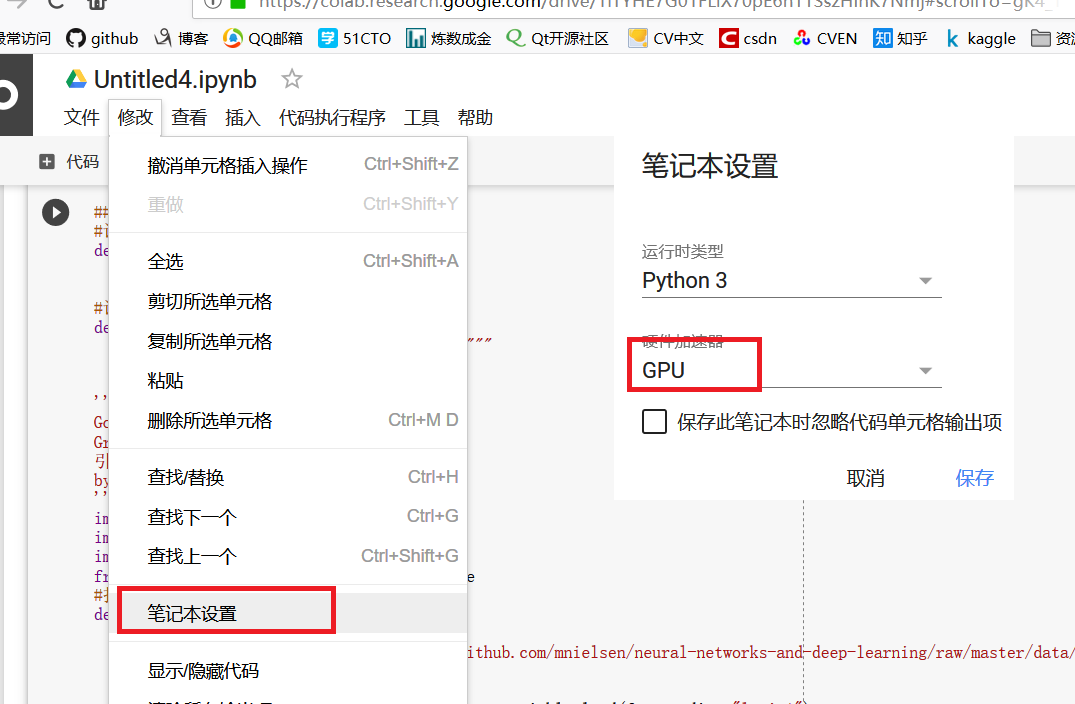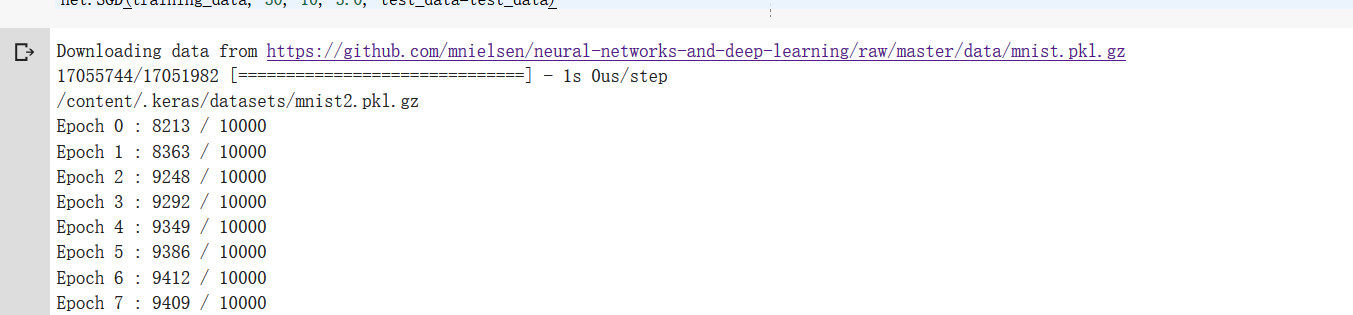# （3网络化部署）自己动手，编写神经网络程序，解决Mnist问题，并网络化部署

+关注继续查看

Colaboratory 是一款研究工具，用于进行机器学习培训和研究。它是一个 Jupyter 笔记本环境，不需要进行任何设置就可以使用。

Jupyter Notebook（此前被称为 IPython notebook）是一个交互式笔记本。

Anaconda中自己带有Jupyter，启动后新建页面（具体内容可以参考相关资料）from keras.utils.data_utils import get_filepath='mnist.npz'path = get_file(path,origin='https://s3.amazonaws.com/img-datasets/mnist.npz',file_hash='8a61469f7ea1b51cbae51d4f78837e45')print(path)#打开Mnist数据def load_data():    path='mnist2.pkl.gz'    path = get_file(path,origin='https://github.com/mnielsen/neural-networks-and-deep-learning/raw/master/data/mnist.pkl.gz')    print(path)    f = gzip.open(path, 'rb')    training_data, validation_data, test_data = pickle.load(f, encoding="latin1")

 # %load D:/dl4cv/GoNetwork/GoNetwork.py# %load network.py"""network.py~~~~~~~~~~IT WORKSA module to implement the stochastic gradient descent learningalgorithm for a feedforward neural network.  Gradients are calculatedusing backpropagation.  Note that I have focused on making the codesimple, easily readable, and easily modifiable.  It is not optimized,and omits many desirable features.jsxyhelu添加了适当的中文注释"""#### Libraries# Standard libraryimport random# Third-party librariesimport numpy as npclass GoNetwork(object):    def __init__(self, sizes):        """size代表的是网络的分层结构，比如[2, 3, 1]        The list sizes contains the number of neurons in the        respective layers of the network.  For example, if the list        was [2, 3, 1] then it would be a three-layer network, with the        first layer containing 2 neurons, the second layer 3 neurons,        and the third layer 1 neuron.  The biases and weights for the        network are initialized randomly, using a Gaussian        distribution with mean 0, and variance 1.  Note that the first        layer is assumed to be an input layer, and by convention we        won't set any biases for those neurons, since biases are only        ever used in computing the outputs from later layers."""        self.num_layers = len(sizes) #层数        self.sizes = sizes #每层size        self.biases = [np.random.randn(y, 1) for y in sizes[1:]]   #随机生成子节点        # net.weights 是⼀个存储着连接第⼆层和第三层神经元权重的 Numpy 矩阵。        self.weights = [np.random.randn(y, x)                        for x, y in zip(sizes[:-1], sizes[1:])]            #前向网络,主要用于测试当前网络                          def feedforward(self, a):        """Return the output of the network if a is input."""        for b, w in zip(self.biases, self.weights):            a = sigmoid(np.dot(w, a)+b)        return a    #随机梯度下降算法    def SGD(self, training_data, epochs, mini_batch_size, eta,            test_data=None):        """Train the neural network using mini-batch stochastic        gradient descent.  The training_data is a list of tuples        (x, y) representing the training inputs and the desired        outputs.  The other non-optional parameters are        self-explanatory.  If test_data is provided then the        network will be evaluated against the test data after each        epoch, and partial progress printed out.  This is useful for        tracking progress, but slows things down substantially."""        training_data = list(training_data)        n = len(training_data)        if test_data:            test_data = list(test_data)            n_test = len(test_data)        #⾸先随机地将训练数据打乱        for j in range(epochs):            random.shuffle(training_data)            #再将它分成多个适当⼤⼩的⼩批量数据            mini_batches = [                training_data[k:k+mini_batch_size]                for k in range(0, n, mini_batch_size)]            #最主要的一行代码            for mini_batch in mini_batches:                self.update_mini_batch(mini_batch, eta)            if test_data:                print("Epoch {} : {} / {}".format(j,self.evaluate(test_data),n_test))            else:                print("Epoch {} complete".format(j))    #根据单次梯度下降的迭代更新⽹络的权重和偏置    def update_mini_batch(self, mini_batch, eta):        """Update the network's weights and biases by applying        gradient descent using backpropagation to a single mini batch.        The mini_batch is a list of tuples (x, y), and eta        is the learning rate."""        nabla_b = [np.zeros(b.shape) for b in self.biases]        nabla_w = [np.zeros(w.shape) for w in self.weights]        for x, y in mini_batch:            delta_nabla_b, delta_nabla_w = self.backprop(x, y)            nabla_b = [nb+dnb for nb, dnb in zip(nabla_b, delta_nabla_b)]            nabla_w = [nw+dnw for nw, dnw in zip(nabla_w, delta_nabla_w)]        self.weights = [w-(eta/len(mini_batch))*nw                        for w, nw in zip(self.weights, nabla_w)]        self.biases = [b-(eta/len(mini_batch))*nb                       for b, nb in zip(self.biases, nabla_b)]    #反向传播就是一种快速计算代价函数梯度的方法，也就是计算delta的一种方法    def backprop(self, x, y):        """Return a tuple (nabla_b, nabla_w) representing the        gradient for the cost function C_x.  nabla_b and        nabla_w are layer-by-layer lists of numpy arrays, similar        to self.biases and self.weights."""        nabla_b = [np.zeros(b.shape) for b in self.biases]        nabla_w = [np.zeros(w.shape) for w in self.weights]        # feedforward        activation = x        activations = [x] # list to store all the activations, layer by layer        zs = [] # list to store all the z vectors, layer by layer        for b, w in zip(self.biases, self.weights):            z = np.dot(w, activation)+b            zs.append(z)            activation = sigmoid(z)            activations.append(activation)        # backward pass        delta = self.cost_derivative(activations[-1], y) * sigmoid_prime(zs[-1]) #bp1        nabla_b[-1] = delta  #bp3        nabla_w[-1] = np.dot(delta, activations[-2].transpose())  #bp4        # Note that the variable l in the loop below is used a little        # differently to the notation in Chapter 2 of the book.  Here,        # l = 1 means the last layer of neurons, l = 2 is the        # second-last layer, and so on.  It's a renumbering of the        # scheme in the book, used here to take advantage of the fact        # that Python can use negative indices in lists.        for l in range(2, self.num_layers):            z = zs[-l]            sp = sigmoid_prime(z)            delta = np.dot(self.weights[-l+1].transpose(), delta) * sp #bp2,注意这里的+1，其实是计算了下一层了            nabla_b[-l] = delta            nabla_w[-l] = np.dot(delta, activations[-l-1].transpose())        return (nabla_b, nabla_w)    #evaluate评价函数    def evaluate(self, test_data):        """Return the number of test inputs for which the neural        network outputs the correct result. Note that the neural        network's output is assumed to be the index of whichever        neuron in the final layer has the highest activation."""        test_results = [(np.argmax(self.feedforward(x)), y)                        for (x, y) in test_data]        return sum(int(x == y) for (x, y) in test_results)    #cost代价函数    def cost_derivative(self, output_activations, y):        """Return the vector of partial derivatives \partial C_x /        \partial a for the output activations."""        return (output_activations-y)#########helper函数#########计算sigmoid，这个函数来自定义def sigmoid(z):    """The sigmoid function."""    return 1.0/(1.0+np.exp(-z))#计算sigmoid的导数，这个函数可以被证明def sigmoid_prime(z):    """Derivative of the sigmoid function."""    return sigmoid(z)*(1-sigmoid(z))'''GoDateSets:GreenOpen 系列引入数据集by:jsxyhelu 2018/3/31'''import pickleimport gzipimport numpy as npfrom keras.utils.data_utils import get_file#打开Mnist数据def load_data():    path='mnist2.pkl.gz'    path = get_file(path,origin='https://github.com/mnielsen/neural-networks-and-deep-learning/raw/master/data/mnist.pkl.gz')    print(path)    f = gzip.open(path, 'rb')    training_data, validation_data, test_data = pickle.load(f, encoding="latin1")    f.close()    return (training_data, validation_data, test_data)#对读取的数据进行重新封装def load_data_wrapper():    tr_d, va_d, te_d = load_data()    training_inputs = [np.reshape(x, (784, 1)) for x in tr_d]    training_results = [vectorized_result(y) for y in tr_d]    training_data = zip(training_inputs, training_results)    validation_inputs = [np.reshape(x, (784, 1)) for x in va_d]    validation_data = zip(validation_inputs, va_d)    test_inputs = [np.reshape(x, (784, 1)) for x in te_d]    test_data = zip(test_inputs, te_d)    return (training_data, validation_data, test_data)#返回OneHot图def vectorized_result(j):    e = np.zeros((10, 1))    e[j] = 1.0    return eimport numpy as npimport randomtraining_data, validation_data, test_data = load_data_wrapper()training_data = list(training_data)  net = GoNetwork([784, 30, 10])net.SGD(training_data, 30, 10, 3.0, test_data=test_data)|
5月前
|

89 0
|
5月前
|

129 0
|
5月前
|

LabVIEW开放神经网络交互工具包【ONNX】，大幅降低人工智能开发门槛，实现飞速推理

63 0
|
5月前
|

127 0
|
6月前
|

2、从零开始构建基于ChatGPT的嵌入式(Embedding)本地医疗客服问答机器人模型（看完就会，看到最后有惊喜）

981 0
|
11月前
|

1197 4|
11月前
|

whylogs工具库的工业实践！机器学习模型流程与效果监控 ⛵

785 2|

2770 0
|

2019阿里云峰会·上海开发者大会于7月24日盛大开幕，本次峰会与未来世界的开发者们分享开源大数据、IT基础设施云化、数据库、云原生、物联网等领域的技术干货， 共同探讨前沿科技趋势。本文整理自开源大数据专场中阿里巴巴资深技术专家李永先生的精彩演讲，将为大家分享AliGraph：大规模图神经网络平台。
7889 0
|

3149 0